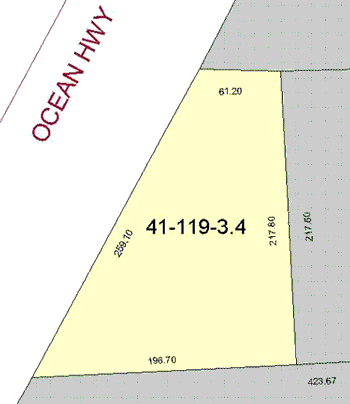Quandaries and Queries I have a problem. I am interested in purchasing a piece of property which has the following dimensions. It is 259.10 Feet on the north side x 61.20 Feet on the east side x 217.80 Feet on the south side x 196.70 Feet on the west side. It looks like an irregular rectangle shape. How do you figure out the square footage on this irregular sized property, and what is the correct square footage answer. I have attached a survey map. Lea Hi Lea, The lengths of the four sides is not enough information to calculate the area but the survey map, if I am reading it correctly, allows me to find the area.The map doesn't say so explicitly but I assume that the dimension, 217.5 feet, is the perpendicular distance between the two horizontal boundaries.If this is the correct intrepetation then I get the area to be 28046.6 square feet which is 0.644 acres. Harley Go to Math Central